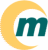s i s t e m a   o p e r a c i o n a l   m a g n u x   l i n u x ~/ · documentaçăo · suporte · sobre

Next Previous Contents

## 4. Verification Script

This is a Korn shell script to verify beautifier program. Requires "pdksh*.rpm" from Linux 'contrib' cdrom. Save this file as 'text' file and chmod a+rx on it. You can re-write this shell script in PERL so that you can use it on Window 95/NT or MSDOS. Uncomment the PRGM variable to point to bcpp, cb or indent

#!/bin/ksh

# Verification program to check C++ Beautifiers 'bcpp', 'indent' or cb
############################################################
# The copyright policy is GNU/GPL.
# Author: Al Dev (Alavoor Vasudevan) alavoor@yahoo.com
############################################################

check_beautify_now()
{
# Remove all the temp files....
\rm -f ${TMP_FILE} \rm -f${TMP_CPPFILE}*.*

FNAME=$1 if [ ! -f${FNAME} ]; then
print "\nError: The file ${FNAME} does not exist!!. Aborting now ...." exit fi \cp -f${FNAME} ${TMP_CPPFILE}.cpp${COMPILER} -c ${TMP_CPPFILE}.cpp if [ ! -f${TMP_CPPFILE}.o ]; then
print "Fatal Error: Failed to compile ${FNAME}. Aborting now... " exit fi \mv -f${TMP_CPPFILE}.o ${TMP_CPPFILE}_orig.o aa=basename$PRGM
print "\nRunning, verifying $aa on${FNAME}"
${PRGM}${TMP_CPPFILE}.cpp
${COMPILER} -c${TMP_CPPFILE}.cpp
\rm -f $TMP_FILE diff${TMP_CPPFILE}.o ${TMP_CPPFILE}_orig.o 1>$TMP_FILE 2>> $TMP_FILE result="" result=wc -c$TMP_FILE | awk '{print $1}'  if [ "$result" = "0" ]; then
print "Success!! Beautifier $aa is working properly!!\n" else print "Fatal Error: Something wrong!! Beautifier is not working!!" exit fi #${COMPILER} -S ${TMP_CPPFILE}.cpp # diff${TMP_CPPFILE}.s ${TMP_CPPFILE}_orig.s # Remove all the temp files.... \rm -f${TMP_FILE}
\rm -f ${TMP_CPPFILE}*.* } ########## Main of program begins here ##################3 #PRGM=/usr/bin/bcpp #PRGM=/usr/bin/cb PRGM=/usr/bin/indent COMPILER=/usr/bin/g++ TMP_FILE=beautify.tmp TMP_CPPFILE=beautify-tmp_cppfile print -n "Enter the C++ file name <default is *.cpp> : " read ans if [ "$ans" = "" -o "$ans" = " " ]; then ans="ALL" else FILENAME=$ans
fi

# Remove all the temp files....
\rm -f ${TMP_FILE} \rm -f${TMP_CPPFILE}*.*

if [ "$ans" != "ALL" ]; then check_beautify_now${FILENAME}
else
ls *.cpp |